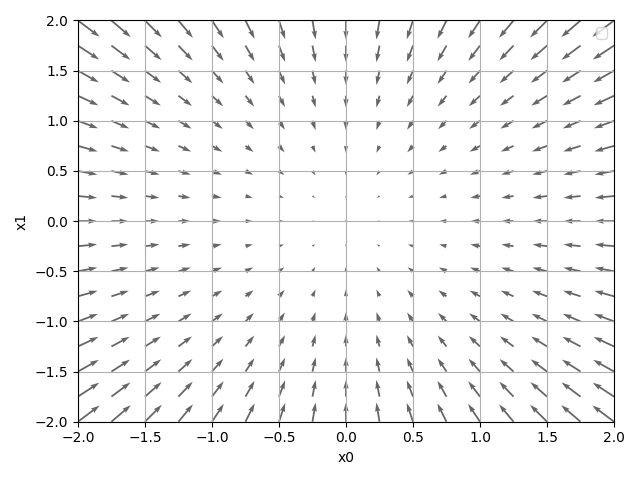# 从零开始的深度学习 Ch2

## Neural Networks learn by...?

Posted by R1NG on January 8, 2021 Viewed Times

# 损失函数和梯度下降法

[注]

## 2. 损失函数和小批量学习法

1. 损失函数非常灵敏, 即使权值中某一个参数出现了微小变化也可能导致损失函数值的改变, 这和 “神经网络的识别正确率” 这一参数不同.
2. 损失函数的变化是连续的, 其导数在定义域上不为 $0$, 以它的导数作为优化方向的指标非常可靠.

1. 均方误差 (Mean Squared Error)

$E = \frac{1}{2}\sum_{k}(y_k - t_k)^2$

其中, $y_k$ 表示神经网络的输出, $t_k$ 表示监督数据 (测试数据), $k$ 是数据的维度.

均方误差的 Python 实现如下:

1
2
def mean_squared_error(y, t):
return 0.5 * np.sum((y-t)**2)


2. 交叉熵误差 (Cross Entropy Error)

$E = -\sum_{k}t_k\ln{(y_k)}$

其中, $y_k$ 为神经网络的输出, $t_k$ 为正确解标签, 且解标签中, 只有正确的值为 $1$, 其余的均为 $0$.

值得注意的是, 在交叉熵误差公式中, 实际上只会计算正确解标签的输出的自然对数. 也就是说, 交叉熵误差是由正确解标签所对应的输出结果决定的.

交叉熵误差的 Python 实现如下:

1
2
3
def cross_entropy_error(y, t):
delta = 1e-7
return -np.sum(t * np.log(y + delta))   # prevent np.log(0) happen


1
2
3
4
5
train_size = x_train.shape
batch_size = 10


## 3. 数值微分和梯度下降法

1
2
3
4
5
6
7
8
9
10
11
12
13
14
15
16
17
h = 1e-4 # 0.0001

for idx in range(x.size):
tmp_val = x[idx]
x[idx] = tmp_val + h
fxh1 = f(x) # f(x+h)

x[idx] = tmp_val - h
fxh2 = f(x) # f(x-h)

grad[idx] = (fxh1 - fxh2) / (2*h)
x[idx] = tmp_val # 还原值1. 梯度所指示的方向是函数值减小最多的方向
2. 距离局部最优点最远, 梯度值越大. (体现在箭头的长度上)

1
2
3
4
5
6
7
8
9
10
11
12
13
14
15

# Args:
#   f: function to be learned (optimized)
#   init_x: initial value
#   lr: learning rate
#   step_num: the number of iterations (learn how many times)

x = init_x

for i in range(step_num):

return x


[例]

$\frac{\partial(L)}{\partial(W)} = \begin{pmatrix} \frac{\partial(L)}{\partial(w_{11})}, \frac{\partial(L)}{\partial(w_{12})}, \frac{\partial(L)}{\partial(w_{13})} \\ ~~~ \\\frac{\partial(L)}{\partial(w_{21})}, \frac{\partial(L)}{\partial(w_{22})}, \frac{\partial(L)}{\partial(w_{23})}\end{pmatrix}$

1
2
3
4
5
6
7
8
9
10
11
12
13
class simpleNet:
def __init__(self):
self.W = np.random.randn(2,3)

def predict(self, x):
return np.dot(x, self.W)

def loss(self, x, t):
z = self.predict(x)
y = softmax(z)
loss = cross_entropy_error(y, t)

return loss


## 4. 随机梯度下降法的实现

1. 从训练数据中随机抽出一部分, 称其为 mini-batch. 学习的目标是优化参数, 尽可能地使 mini-batch 的损失函数最小化.
2. 求出各个权重参数的梯度
3. 将权重参数沿梯度方向进行更新
4. 重复上述步骤, 直到重复次数达到设定值.

1
2
3
4
5
6
7
8
9
10
11
12
13
14
15
16
17
18
19
20
21
22
23
24
25
26
27
28
29
30
31
32
33
34
35
36
37
38
39
40
41
42
43
44
45
46
47
48
49
50
51
52
53
54
55
56
57
58
59
60
61
62
63
64
65
66
67
68
69
70
71
72
73
74
75
76
class TwoLayerNet:

def __init__(self, input_size, hidden_size, output_size, weight_init_std=0.01):
# 初始化权重

self.params = {}
self.params['W1'] = weight_init_std * np.random.randn(input_size, hidden_size)
self.params['b1'] = np.zeros(hidden_size)
self.params['W2'] = weight_init_std * np.random.randn(hidden_size, output_size)
self.params['b2'] = np.zeros(output_size)

def predict(self, x):
W1, W2 = self.params['W1'], self.params['W2']
b1, b2 = self.params['b1'], self.params['b2']

a1 = np.dot(x, W1) + b1
z1 = sigmoid(a1)
a2 = np.dot(z1, W2) + b2
y = softmax(a2)

return y

# x:输入数据, t:监督数据

def loss(self, x, t):
y = self.predict(x)

return cross_entropy_error(y, t)

def accuracy(self, x, t):
y = self.predict(x)
y = np.argmax(y, axis=1)
t = np.argmax(t, axis=1)

accuracy = np.sum(y == t) / float(x.shape)
return accuracy

# x:输入数据, t:监督数据

loss_W = lambda W: self.loss(x, t)

W1, W2 = self.params['W1'], self.params['W2']
b1, b2 = self.params['b1'], self.params['b2']

batch_num = x.shape

# forward

a1 = np.dot(x, W1) + b1
z1 = sigmoid(a1)
a2 = np.dot(z1, W2) + b2
y = softmax(a2)

# backward

dy = (y - t) / batch_num